# Joins in Tableau: How to Join Data in Tableau 2021

Performing join in Tableau is the most crucial functions, and we can join two or more tables having standard fields. Relationships are a dynamic, flexible way to combine data from multiple tables for analysis. In Tableau, when we work with massive datasets, it is natural that datasets will have various tables with different data fields.

## Why joins are useful in Tableau

If you are working with real-time databases, datasets, and data warehouses, you know that can not fit in a single table; you need multiple tables to store the data. This means that the data does not reside on a single table. Instead, there are different tables. However, some tables might have standard fields. We can join such tables using columns that are common or related.

Joins in Tableau play an essential role in designing reports. In real-time, We usually create reports using the database or data warehouse, and we all know that the data present in the database is in normalized form(like DBMS).

So, we can’t generate reports using the single table (mostly not, as per the business perspective).

For example. Let’s say and we have two tables or data sets.

1. Orders
2. Products

The Orders table has OrderID, OrderName, PrductID, and the Products table has ProductID, ProductName, ProductPrice.

Now, when the user places an order, then inside the order table, ProductID is stored.

That means we have connected or joined a table using ProductID.

If you have studied SQL, then you remember that inside the Orders table, OrderID is a primary key, and ProductID is a foreign key.

In the Products table, ProductID is the primary key. So, we can join the two tables using ProductID.

## Characteristics of Joins in Tableau

Joins are a more static way to combine data. Joins must be defined between physical tables upfront before analysis and can’t be changed without impacting all sheets using that data source.

Joined tables are always merged into a single table. As a result, sometimes joined data is missing unmatched values or duplicates aggregated values.

1. Joins are displayed with Venn diagram icons between physical tables.
2. Joins require you to select join types and join clauses.
3. Joined physical tables are merged into a single logical table with a fixed combination of data.
4. Joins may drop unmatched measure values.
5. Joins may duplicate aggregate values when fields are at different levels of detail.
6. Joins can be defined using calculated fields and inequality operators.
7. Support scenarios that require a single table of data, such as extract filters and row-level security.

## Rules to Perform a Join in Tableau

1. The tables that you are joining must have at least one common column or field.
2. Make sure that the data types of the tables you are joining are the same. If you create the join of tables with different data types, then the Join will break.
3. Define the right join type and join clause for your Join operation to optimize the performance of Tableau with the data. If the join type you select is not valid for the tables, the Join formed will not be accurate and won’t give you efficient data analysis results.

## How to specify Joins in Tableau

You can define the joins between tables in the physical layer of the data source. First, double-click the logical table to go to the Join/Union canvas in the physical layer and add joins or unions.

Every top-level logical table contains at least one physical table.

Open the logical table to view, edit, or create joins between its physical tables.

Right-click the logical table, and then click Open. Or, double-click the table to open it.

When you create a data source, it has two layers.

1. Logical layer
2. Physical layer

The top-level layer is the logical layer of the data source. You combine data between tables in the logical layer using relationships.

The next layer is the physical layer of the data source. You combine data between tables at the physical layer using joins.

Okay, now let’s see some practical examples.

## Steps to Join Data in Tableau

In this tutorial, I am using Tableau Desktop 2021.2 version, and it is the latest version right now. If you are using the older version of Tableau, then visually performing the joins might be different than my version because, in this version, there are lots of updates. So, this is the latest tutorial on performing joins in Tableau.

In this example, we will go step by step to join data in Tableau.

## Step 1: Prepare Datasets

In this example, we will connect Tableau to Microsoft Excel. If you don’t know how to connect Data Source to Tableau, check out connect Data Source to Tableau tutorial.

Now, we will have two Datasets or Tables if you prefer. You can download both Datasets from here: Datasets.zip.

In these Datasets, there are two Excel sheets or Datasets or Tables.

1. Orders.xlsx
2. Products.xlsx

As we have discussed earlier, we will use these two Datasets to join Tableau.

Now, we have our Datasets ready. Next, move to the next step.

## Step 2: Open two Datasets in Tableau

If you have not installed Tableau on Mac, check out how to install Tableau desktop on mac tutorial. You can also use Windows or Linux for this article.

Now, connect the Dataset one by one.

Let’s connect the Products.xlsx Dataset to Tableau.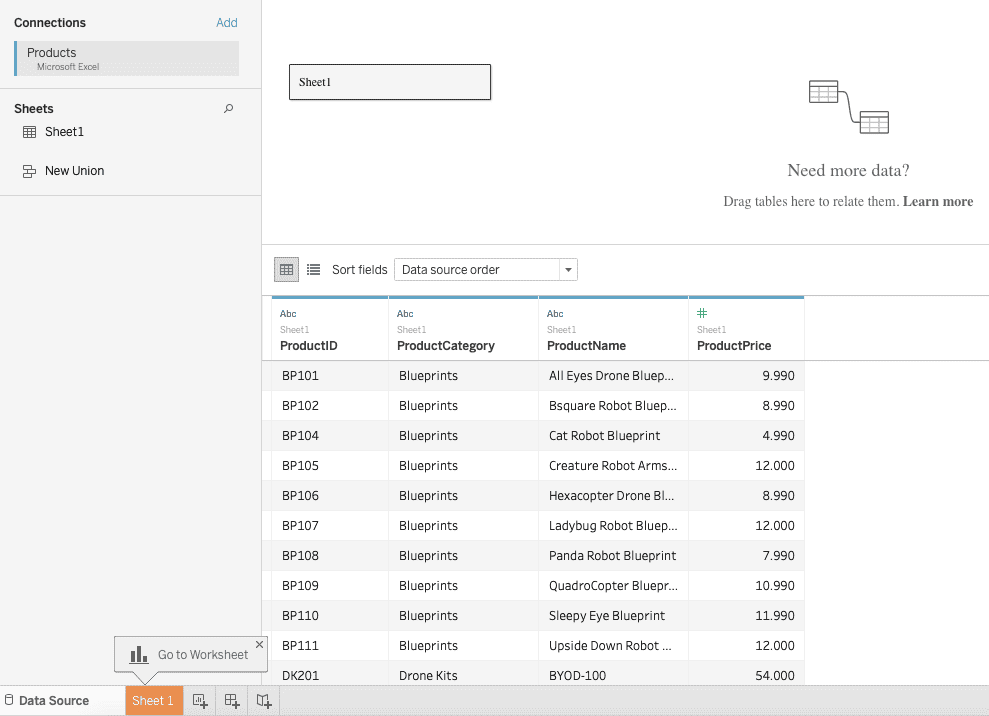Okay, so we have loaded the data of Products in Tableau.

In the above screenshot in the left pane, there is an add button; please click on that button to create a second connection with the Orders.xlsx dataset. So, let’s connect the Orders.xlsx Dataset to Tableau.

So, you will see something like this.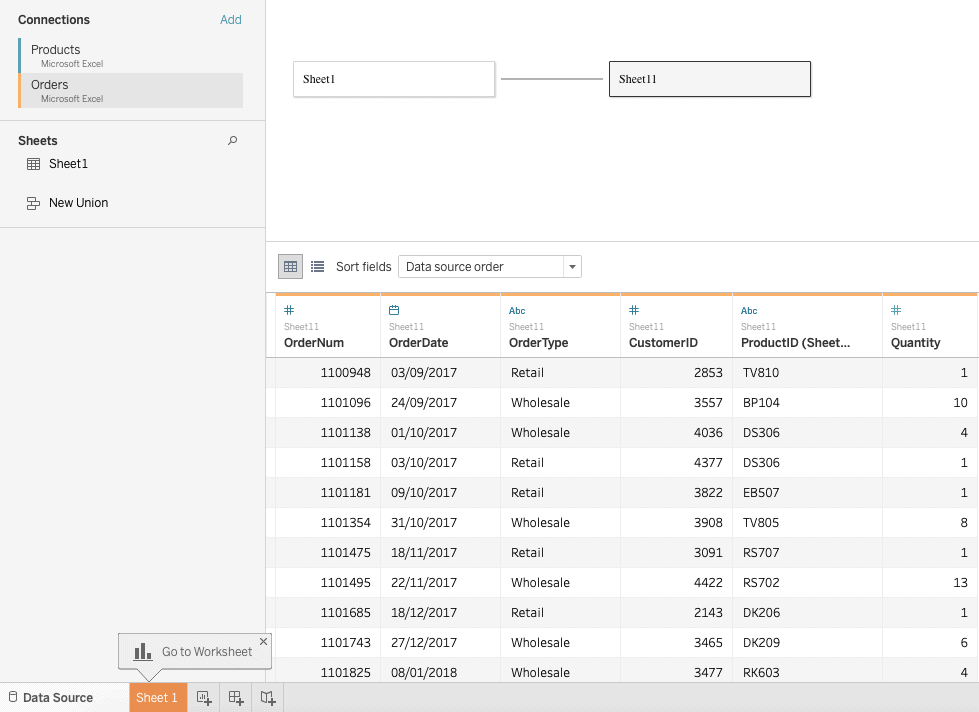In the above figure, in the logical layer, which is the top of the layer, we can see two sheets.

1. Sheet1(Products Dataset)
2. Sheet11(Orders Dataset)

If you click on Sheet1, then you will see the Products Data, and if you click on Sheet11, then you will see Orders data.

Now, we will define the Joins between them in the Physical layer.

Remember, you can not define a join in the Logical layer. Right now, we are in the logical layer.

To create a join, we need to go to the Physical layer.

## Step 3: Join Data in the Physical layer.

On the right side, under Sheet1, there is Sheet1 is selected. Now Double Click on Sheet1 in the Logical layer, and you will go to the Physical layer.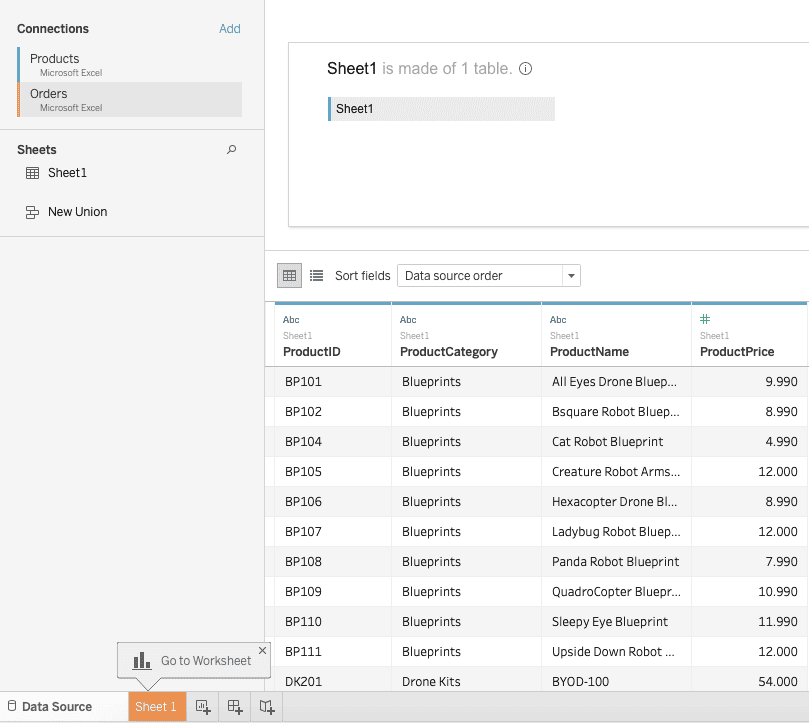Now, this is your current screen.

If you see the left-hand side pane, there is a section called Sheets, and under that section, there is one item called Sheet1.

Now, click and drag Sheet1 and drop to the physical layer.

You will see something like this below.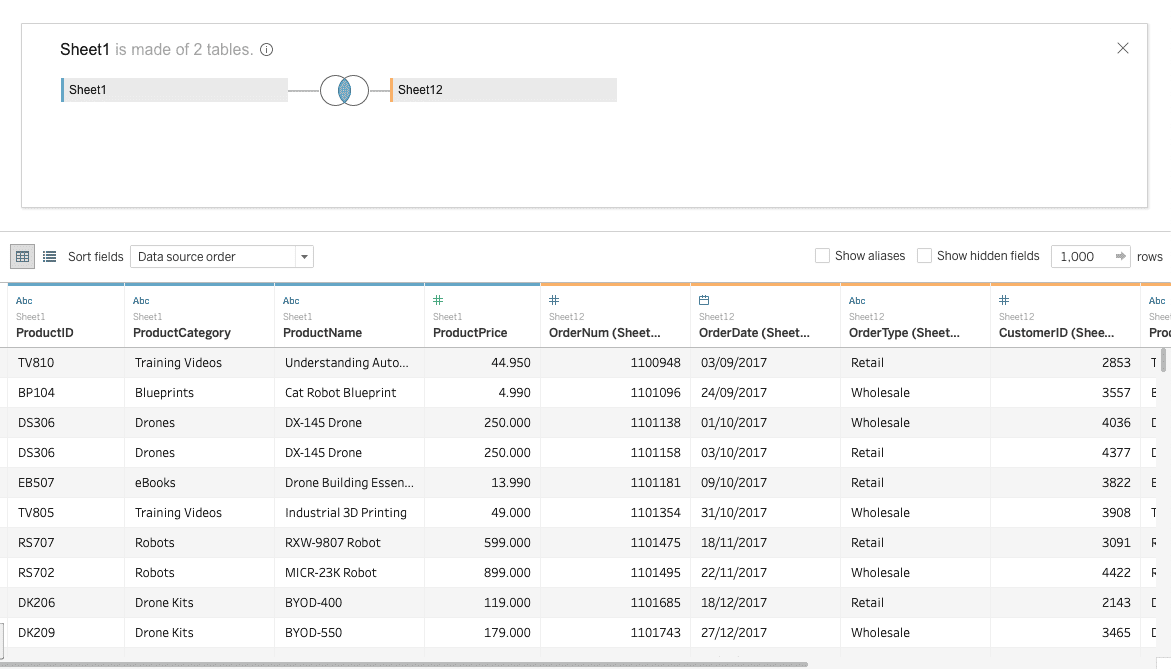Now, you can see that the Join is created between two tables, and you can see the Venn diagram of the inner Join. Here, Sheet12 is the result table of the inner Join.If you hover over the Venn diagram, you will see that the table is joined using ProductID.

If you click on the Venn diagram, then you will see something like this.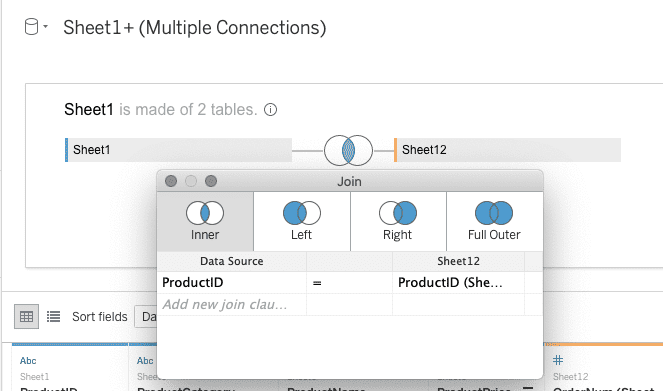This is an inner join.

When the inner join is formed between two tables (that is, left and right), then the resulting or joined table contains only the values or columns that are common between those tables.

In the above small window, you can select the different joins, and the table’s data will change according to selected joins.

If you select a particular join, then a kind of query or operation will be performed, and it will change the result table. The Sheet12 is the result table here.

In this example, Orders and Products are two physical tables, and Sheet12 is the logical table formed due to the inner Join of two physical tables. We have joined the table using ProductID.

## Types of Join in Tableau

1. Inner Join
2. Right join
3. Left Join
4. Full outer Join

### Left Join

If the left join is formed between two tables, then the resulting or joined table contains all the values from the left table and only matches or common values from the right table. If there are no joins or matches in the right table, null values are present in the data grid.

Let’s select left join in our example.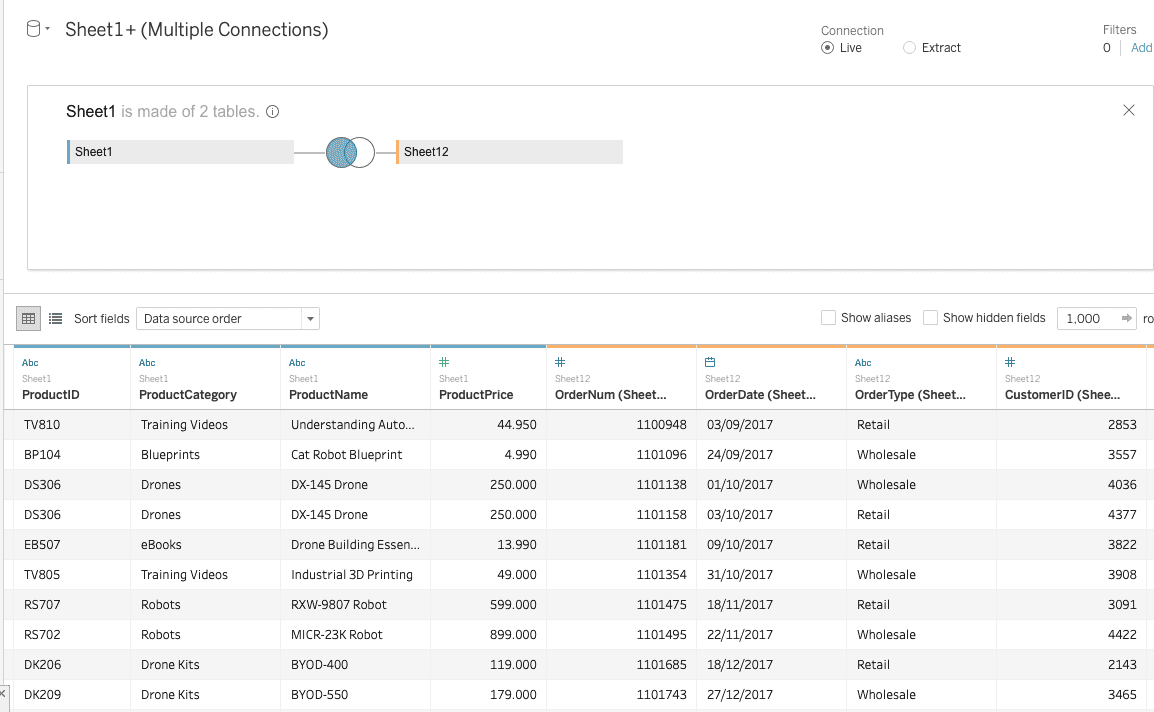### Right Join

When the right Join is formed between two tables, the joined or resulting table contains all the values of the right table and only the matching values from the left table. The values that do not find a match in the left table are left as null in the resulting table.

Let’s form right join in our example. Select write join in the small window.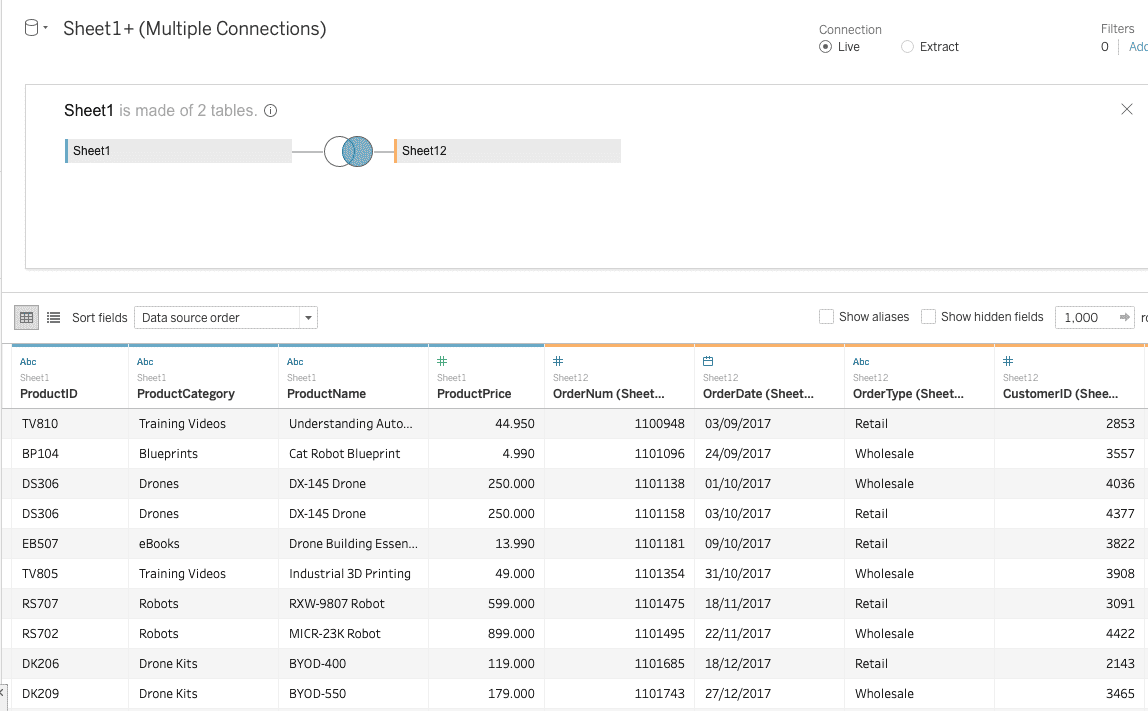### Full Outer Join

When a full outer join is formed between two tables, the joined or resulting table contains all the data values from both the left and right tables. Also, the values that do not match in both the tables are shown as null in the resulting table.

Let’s form Full outer Join in our example.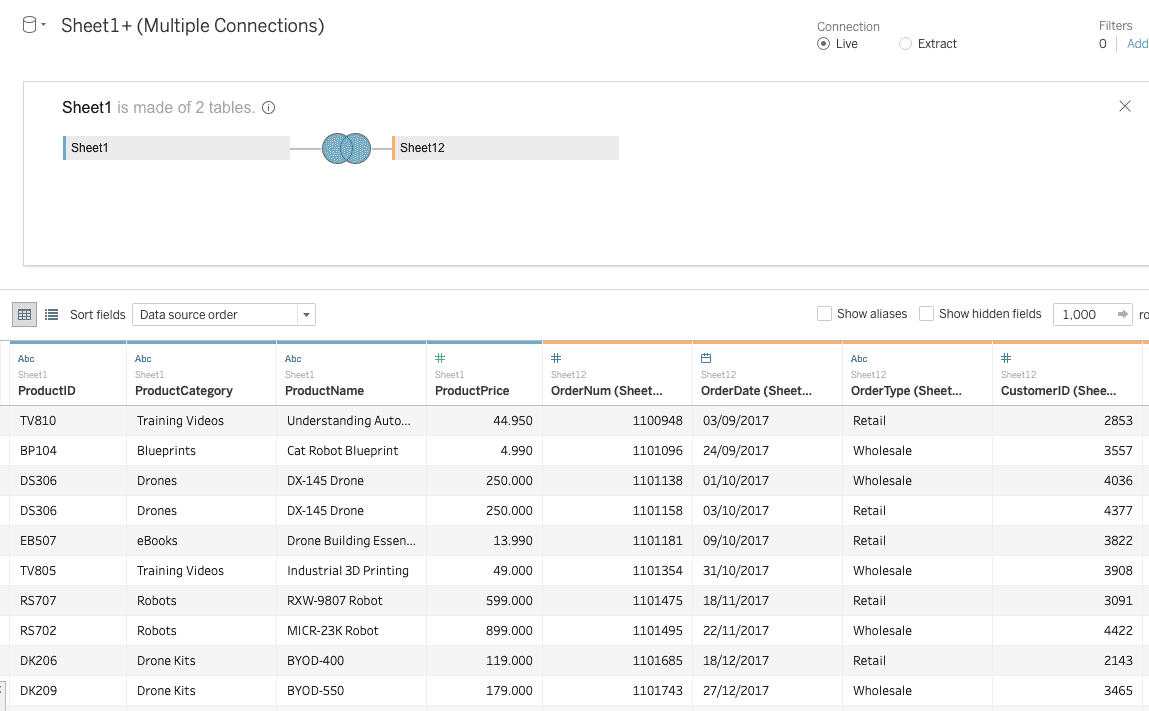## Conclusion

In this tutorial, we have seen what is Join in Tableau and why joins are so important. Then we have seen characteristics of Join, types of joins, and perform the different joins on Tableau.

### 1 thought on “Joins in Tableau: How to Join Data in Tableau 2021”

1.Nicee post. I learn somjething new and challenging on blogs I stumbleupon everyday.

It’s always exciting too read content from other writers and practice something from other websites.

оформление фасадов decoration of buildings